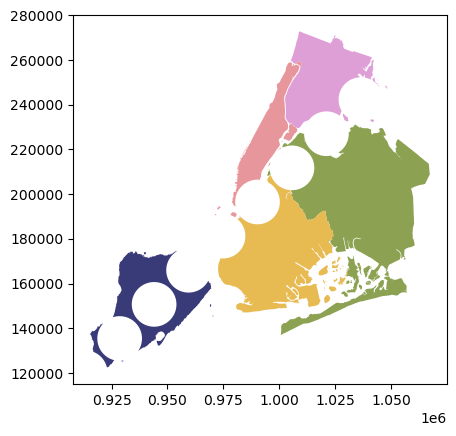Note

# Overlays#

Spatial overlays allow you to compare two GeoDataFrames containing polygon or multipolygon geometries and create a new GeoDataFrame with the new geometries representing the spatial combination and merged properties. This allows you to answer questions like

What are the demographics of the census tracts within 1000 ft of the highway?

The basic idea is demonstrated by the graphic below but keep in mind that overlays operate at the dataframe level, not on individual geometries, and the properties from both are retained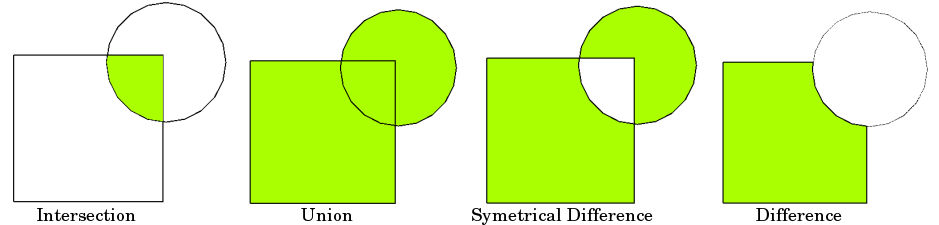Now we can load up two GeoDataFrames containing (multi)polygon geometries…

:

%matplotlib inline
from shapely.geometry import Point
from geopandas.tools import overlay
from geodatasets import get_path

# NYC Boros
zippath = get_path("nybb")

# Generate some circles
b = [int(x) for x in polydf.total_bounds]
N = 10
polydf2 = GeoDataFrame(
[
{"geometry": Point(x, y).buffer(10000), "value1": x + y, "value2": x - y}
for x, y in zip(
range(b, b, int((b - b) / N)),
range(b, b, int((b - b) / N)),
)
]
)

ERROR 1: PROJ: proj_create_from_database: Open of /home/docs/checkouts/readthedocs.org/user_builds/geopandas/conda/stable/share/proj failed


The first dataframe contains multipolygons of the NYC boros

:

polydf.plot()

:

<Axes: >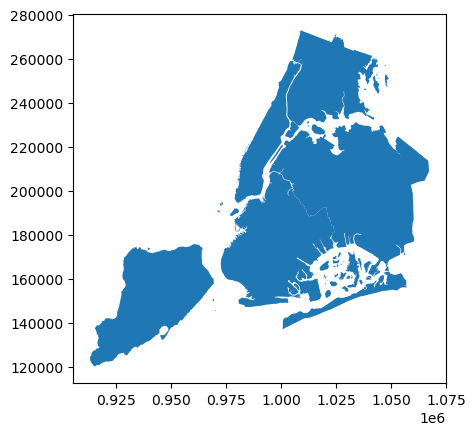And the second GeoDataFrame is a sequentially generated set of circles in the same geographic space. We’ll plot these with a different color palette.

:

polydf2.plot(cmap="tab20b")

:

<Axes: >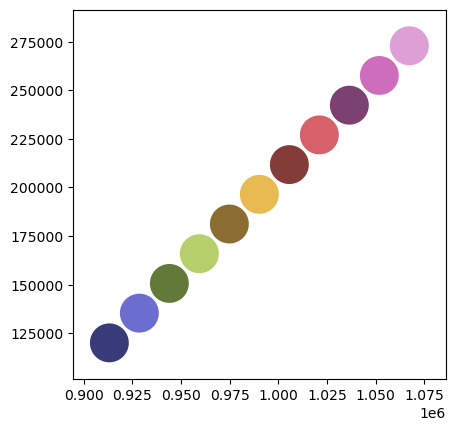The geopandas.tools.overlay function takes three arguments:

• df1

• df2

• how

Where how can be one of:

['intersection',
'union',
'identity',
'symmetric_difference',
'difference']


So let’s identify the areas (and attributes) where both dataframes intersect using the overlay method.

:

newdf = polydf.overlay(polydf2, how="intersection")
newdf.plot(cmap="tab20b")

/home/docs/checkouts/readthedocs.org/user_builds/geopandas/conda/stable/lib/python3.11/site-packages/geopandas/geodataframe.py:2469: UserWarning: CRS mismatch between the CRS of left geometries and the CRS of right geometries.
Use to_crs() to reproject one of the input geometries to match the CRS of the other.

Left CRS: EPSG:2263
Right CRS: None

return geopandas.overlay(

:

<Axes: >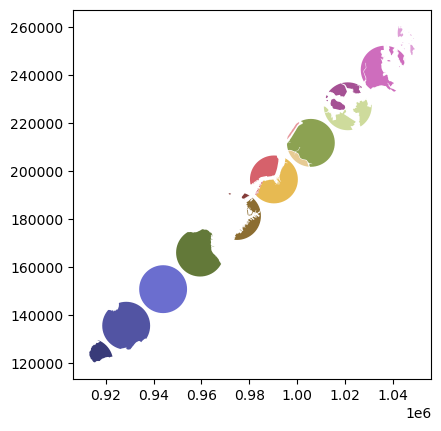And take a look at the attributes; we see that the attributes from both of the original GeoDataFrames are retained.

:

polydf.head()

:

BoroCode BoroName Shape_Leng Shape_Area geometry
0 5 Staten Island 330470.010332 1.623820e+09 MULTIPOLYGON (((970217.022 145643.332, 970227....
1 4 Queens 896344.047763 3.045213e+09 MULTIPOLYGON (((1029606.077 156073.814, 102957...
2 3 Brooklyn 741080.523166 1.937479e+09 MULTIPOLYGON (((1021176.479 151374.797, 102100...
3 1 Manhattan 359299.096471 6.364715e+08 MULTIPOLYGON (((981219.056 188655.316, 980940....
4 2 Bronx 464392.991824 1.186925e+09 MULTIPOLYGON (((1012821.806 229228.265, 101278...
:

polydf2.head()

:

geometry value1 value2
0 POLYGON ((923175.000 120121.000, 923126.847 11... 1033296 793054
1 POLYGON ((938595.000 135393.000, 938546.847 13... 1063988 793202
2 POLYGON ((954015.000 150665.000, 953966.847 14... 1094680 793350
3 POLYGON ((969435.000 165937.000, 969386.847 16... 1125372 793498
4 POLYGON ((984855.000 181209.000, 984806.847 18... 1156064 793646
:

newdf.head()

:

BoroCode BoroName Shape_Leng Shape_Area value1 value2 geometry
0 5 Staten Island 330470.010332 1.623820e+09 1033296 793054 POLYGON ((922861.155 122515.103, 922832.456 12...
1 5 Staten Island 330470.010332 1.623820e+09 1063988 793202 POLYGON ((936924.598 129868.857, 936896.231 12...
2 5 Staten Island 330470.010332 1.623820e+09 1094680 793350 POLYGON ((953966.847 149684.829, 953822.853 14...
3 5 Staten Island 330470.010332 1.623820e+09 1125372 793498 POLYGON ((961460.942 175472.260, 961482.499 17...
4 4 Queens 896344.047763 3.045213e+09 1217448 793942 POLYGON ((1005319.370 201772.684, 1005317.989 ...

Now let’s look at the other how operations:

:

newdf = polydf.overlay(polydf2, how="union")
newdf.plot(cmap="tab20b")

/home/docs/checkouts/readthedocs.org/user_builds/geopandas/conda/stable/lib/python3.11/site-packages/geopandas/geodataframe.py:2469: UserWarning: CRS mismatch between the CRS of left geometries and the CRS of right geometries.
Use to_crs() to reproject one of the input geometries to match the CRS of the other.

Left CRS: EPSG:2263
Right CRS: None

return geopandas.overlay(

:

<Axes: >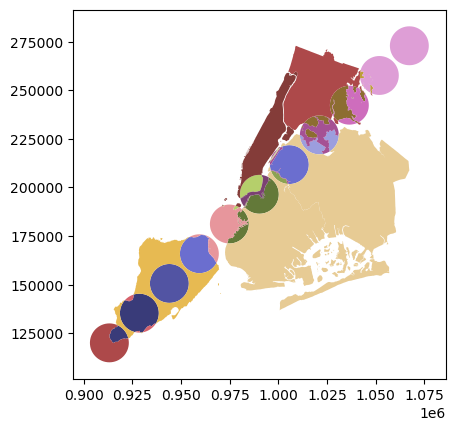:

newdf = polydf.overlay(polydf2, how="identity")
newdf.plot(cmap="tab20b")

/home/docs/checkouts/readthedocs.org/user_builds/geopandas/conda/stable/lib/python3.11/site-packages/geopandas/geodataframe.py:2469: UserWarning: CRS mismatch between the CRS of left geometries and the CRS of right geometries.
Use to_crs() to reproject one of the input geometries to match the CRS of the other.

Left CRS: EPSG:2263
Right CRS: None

return geopandas.overlay(

:

<Axes: >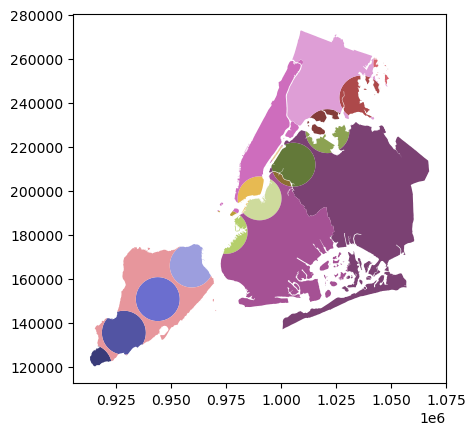:

newdf = polydf.overlay(polydf2, how="symmetric_difference")
newdf.plot(cmap="tab20b")

/home/docs/checkouts/readthedocs.org/user_builds/geopandas/conda/stable/lib/python3.11/site-packages/geopandas/geodataframe.py:2469: UserWarning: CRS mismatch between the CRS of left geometries and the CRS of right geometries.
Use to_crs() to reproject one of the input geometries to match the CRS of the other.

Left CRS: EPSG:2263
Right CRS: None

return geopandas.overlay(

:

<Axes: >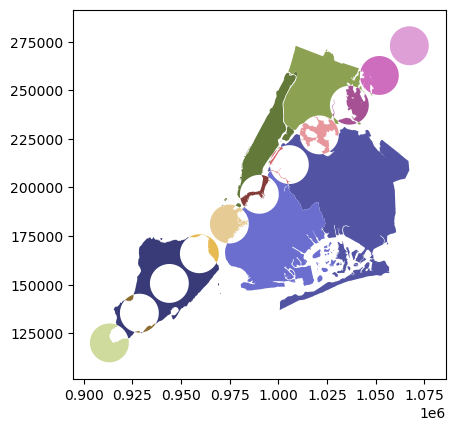:

newdf = polydf.overlay(polydf2, how="difference")
newdf.plot(cmap="tab20b")

/home/docs/checkouts/readthedocs.org/user_builds/geopandas/conda/stable/lib/python3.11/site-packages/geopandas/geodataframe.py:2469: UserWarning: CRS mismatch between the CRS of left geometries and the CRS of right geometries.
Use to_crs() to reproject one of the input geometries to match the CRS of the other.

Left CRS: EPSG:2263
Right CRS: None

return geopandas.overlay(

:

<Axes: >